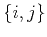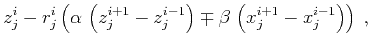Huygens wavefront tracing: A robust alternative to conventional ray tracingNext: Implementation details Up: Sava & Fomel: Huygens Previous: Continuous theory

# A discretization scheme and the Huygens' principle

A natural first-order discretization scheme for equation (5) leads to the difference equation(7)

where the indexcorresponds to the ray parameter,corresponds to the traveltime,,is the increment in time, andis the velocity at thegrid point. It is easy to notice that equation (7) simply describes a sphere (or a circle in two dimensions) with the center atand the radius. This sphere is, of course, the wavefront of a secondary Huygens source.

This observation suggests that we apply the Huygens' principle directly to find an appropriate discretization for equation (6). Let us consider a family of Huygens spheres, centered at the points along the current wavefront. Mathematically, this family is described by an equation analogous to (7), as follows:(8)

Here the ray parameterserves as the parameter that distinguishes a particular Huygens source. According to the Huygens' principle, the next wavefront corresponds to the envelope of the wavefront family. To find the envelop condition, we can simply differentiate both sides of equation (8) with respect to the family parameter. The result takes the form(9)

which is clearly a semidiscrete analog of equation (6). To complete the discretization, we can represent the-derivatives in (9) by a centered finite-difference approximation. This representation yields the scheme(10)

which supplements the previously found scheme (7) for a unique determination of the pointon the-th ray and the-th wavefront. Formulas (7) and (10) define an update scheme, depicted in Figure 1. To fill theplane, the scheme needs to be initialized with one complete wavefront (around the wave source) and two boundary rays.

The solution of system (7-10) has the explicit form(11)(12)

where(13)

and(14)

scheme
Figure 1.
An updating scheme for HWT. Three points on the current wavefront (,, and) are used to advance in thedirection.Figure 2 shows a geometric interpretation of formulas (7) and (10). Formula (10) is clearly a line equation. Thus, the new pointin Figure 2 is defined as one of the two intersections of this line with thesphere, defined by formula (7). It is easy to show geometrically that the newly created ray segmentis orthogonal to the common tangent of spheresand. Within the finite-difference approximation, the common tangent reflects local wavefront behavior.

huygens
Figure 2.
A geometrical updating scheme for HWT in the physical domain. Three points on the current wavefront (,, and) are used to compute the position of thepoint. The bold lines represent equations (7) and (10). The tangent to circleat pointis parallel to the common tangent of circlesand.Huygens wavefront tracing: A robust alternative to conventional ray tracingNext: Implementation details Up: Sava & Fomel: Huygens Previous: Continuous theory

2014-03-11